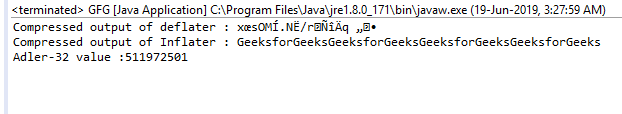# Inflater getAdler() function in Java with examples

The getAdler() function of the Inflater class returns the Adler-32 value of the uncompressed data. Adler-32 is a checksum algorithm which is widely used zlib compression library.

Function Signature:

```public int getAdler()
```

Syntax:

```i.getAdler();
```

Parameter: The function requires no parameter

Return Type: The function returns Integer value which is the Adler-32 value of the uncompressed data.
Exception: The function does not throw any exception

Example 1: use of getAdler() function

 `// Java program to describe the use ` `// of getAdler function ` ` `  `import` `java.util.zip.*; ` `import` `java.io.UnsupportedEncodingException; ` `class` `GFG { ` `    ``public` `static` `void` `main(String args[]) ` `        ``throws` `UnsupportedEncodingException, ` `               ``DataFormatException ` `    ``{ ` ` `  `        ``// compress the data ` ` `  `        ``// deflater ` `        ``Deflater d = ``new` `Deflater(); ` ` `  `        ``// get the text ` `        ``String pattern = ``"GeeksforGeeks"``, text = ``""``; ` ` `  `        ``// generate the text ` `        ``for` `(``int` `i = ``0``; i < ``4``; i++) ` `            ``text += pattern; ` ` `  `        ``// set the input for deflator ` `        ``d.setInput(text.getBytes(``"UTF-8"``)); ` ` `  `        ``// finish ` `        ``d.finish(); ` ` `  `        ``// output bytes ` `        ``byte` `output[] = ``new` `byte``[``1024``]; ` ` `  `        ``// compress the data ` `        ``int` `size = d.deflate(output); ` ` `  `        ``// end ` `        ``d.end(); ` ` `  `        ``// end of compression ` ` `  `        ``// use Inflater to get back the original data ` ` `  `        ``// Inflater ` `        ``Inflater i = ``new` `Inflater(); ` ` `  `        ``// set the input for inflator ` `        ``i.setInput(output); ` ` `  `        ``// output bytes ` `        ``byte` `inflater_output[] = ``new` `byte``[``1024``]; ` ` `  `        ``// uncompress the data ` `        ``int` `org_size = i.inflate(inflater_output); ` ` `  `        ``// output of inflater and deflater ` `        ``System.out.println(``"Compressed output of deflater : "` `                           ``+ ``new` `String(output)); ` `        ``System.out.println(``"Compressed output of Inflater : "` `                           ``+ ``new` `String(inflater_output, ``"UTF-8"``)); ` ` `  `        ``// get Adler-32 value ` `        ``System.out.println(``"Adler-32 value :"` `+ i.getAdler()); ` ` `  `        ``// end ` `        ``i.end(); ` `    ``} ` `} `

Output:Attention reader! Don’t stop learning now. Get hold of all the important Java and Collections concepts with the Fundamentals of Java and Java Collections Course at a student-friendly price and become industry ready.

My Personal Notes arrow_drop_upThird year Department of Information Technology Jadavpur University

If you like GeeksforGeeks and would like to contribute, you can also write an article using contribute.geeksforgeeks.org or mail your article to contribute@geeksforgeeks.org. See your article appearing on the GeeksforGeeks main page and help other Geeks.

Please Improve this article if you find anything incorrect by clicking on the "Improve Article" button below.

Article Tags :
Practice Tags :

Be the First to upvote.

Please write to us at contribute@geeksforgeeks.org to report any issue with the above content.